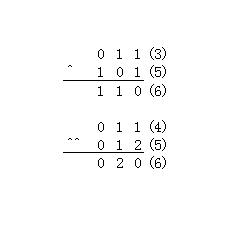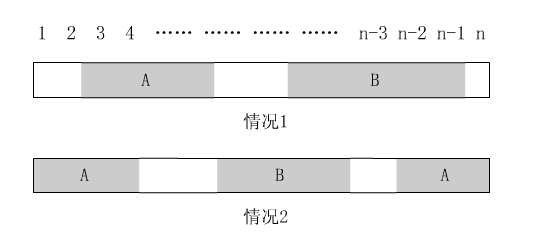### 最新评论：leijiankun 说：膜拜神牛。……css 说：康拓展开和逆康拓展……songrenchu 说：欢迎大家踊……Admin 说：……

# 【有道难题资格赛一】解题报告

## 发表时间：2010-05-31 11:11:34  浏览(3264) 评论(2)

A：另类的异或

【题目叙述】【输入】

A B C
A和C是两个十进制整数，B是一个字符串，由n个^组成
1 <= T <= 100, 0<=A,B<2^30, 1<=n<=1000

【输出】

【样例输入】
2
3 ^ 5
4 ^^ 5

【样例输出】
6
6

【算法分析】

【我的代码】
var i,j,k,l,m,n,p,q,r,t:longint;
num1,num2,ans:array[0..100] of longint;
oup:longint;
c:char;

begin
for i:=1 to t do
begin
p:=0;
while true do
begin
if (c in ['0'..'9']) then p:=p*10+ord(c)-48
else break;
end;
k:=1;
while true do
begin
if (c='^') then break;
end;
while true do
begin
inc(k);
if (c<>'^') then break;
end;
q:=0;
while not eoln do
begin
if (c in ['0'..'9']) then q:=q*10+ord(c)-48;
end;
m:=0;
fillchar(num1,sizeof(num1),0);
fillchar(num2,sizeof(num2),0);
fillchar(ans,sizeof(ans),0);
while (p>0) do
begin
inc(m);
num1[m]:=p mod k;
p:=p div k;
end;
m:=0;
while (q>0) do
begin
inc(m);
num2[m]:=q mod k;
q:=q div k;
end;
for j:=1 to 100 do ans[j]:=(num1[j]+num2[j]) mod k;
for l:=100 downto 1 do if (ans[l]>0) then break;
oup:=0;
for j:=l downto 1 do oup:=oup*k+ans[j];
writeln(oup);
end;
end.

【现场总结】

B：有道搜索框

【题目叙述】【输入】

【输出】

【样例输入】
10
a
ab
hello
that
those
dict
youdao
world
your
dictionary
6
bob
d
dict
dicti
yo
z

【样例输出】
bob
dict dictionary
dict dictionary
dictionary
youdao your
z

【算法分析】

【我的代码】
var i,j,k,l,m,n,p,q,r,t,many,left:longint;
trie:array[0..200000,'a'..'z'] of longint;
have:array[0..200000] of boolean;
s:string;
can:boolean;

procedure ins_trie(s:string);
var g:longint;
begin
p:=0;
for g:=1 to length(s) do
if (trie[p,s[g]]>0) then p:=trie[p,s[g]]
else
begin
inc(many);
trie[p,s[g]]:=many;
p:=many;
end;
have[p]:=true;
end;

procedure dfs(s:string;x:longint);
var c:char;
begin
if (left=0) then exit;
if (have[x]) then
begin
if (left<8) then write(' ');
write(s);dec(left);
end;
for c:='a' to 'z' do
if trie[x,c]>0 then dfs(s+c,trie[x,c]);
end;

begin
many:=0;
fillchar(trie,sizeof(trie),0);
for i:=1 to n do
begin
ins_trie(s);
end;
for i:=1 to q do
begin
p:=0;can:=true;
for j:=1 to length(s) do
if (trie[p,s[j]]=0) then
begin
can:=false;break;
end else p:=trie[p,s[j]];
if (not can) then writeln(s) else
begin
left:=8;
dfs(s,p);writeln;
end;
end;
end.

【现场总结】

C：最大和子序列

【题目叙述】

【输入】

【输出】

【样例输入】
3
3
1 -1 0
4
1 -1 1 -1
5
1 -1 1 -1 1

【样例输出】
1
2
3

【算法分析】【我的代码】
var i,j,k,l,m,n,p,q,r,s,t,dt:longint;
a:array[0..50001] of longint;
left,right,leftmax,rightmax,leftmin,rightmin,leftdt,rightdt:array[0..50001] of longint;
ans:longint;

function more(x,y:longint):longint;
begin
if (x>y) then exit(x) else exit(y);
end;

function less(x,y:longint):longint;
begin
if (x<y) then exit(x) else exit(y);
end;

begin
for dt:=1 to t do
begin
fillchar(left,sizeof(left),0);
fillchar(right,sizeof(right),0);
leftmax:=-maxlongint;leftmin:=maxlongint;
rightmax[n+1]:=-maxlongint;rightmin[n+1]:=maxlongint;
for i:=1 to n do read(a[i]);
for i:=1 to n do
begin
left[i]:=left[i-1]+a[i];
leftmax[i]:=leftmax[i-1];
if (left[i]>leftmax[i]) then leftmax[i]:=left[i];
leftmin[i]:=leftmin[i-1];
if (left[i]<leftmin[i]) then leftmin[i]:=left[i];
end;
for i:=n downto 1 do
begin
right[i]:=right[i+1]+a[i];
rightmax[i]:=rightmax[i+1];
if (right[i]>rightmax[i]) then rightmax[i]:=right[i];
rightmin[i]:=rightmin[i+1];
if (right[i]<rightmin[i]) then rightmin[i]:=right[i];
end;
ans:=-maxlongint;
for i:=1 to n-1 do
if (leftmax[i]+rightmax[i+1]>ans) then ans:=leftmax[i]+rightmax[i+1];

leftdt:=left;
for i:=2 to n do
begin
leftdt[i]:=leftdt[i-1];
if (left[i]-leftmin[i-1]>leftdt[i]) then leftdt[i]:=left[i]-leftmin[i-1];
end;
rightdt[n]:=right[n];
for i:=n-1 downto 1 do
begin
rightdt[i]:=rightdt[i+1];
if (right[i]-rightmin[i+1]>rightdt[i]) then rightdt[i]:=right[i]-rightmin[i+1];
end;
for i:=1 to n-1 do
begin
p:=more(left[i],leftdt[i]);
q:=more(right[i+1],rightdt[i+1]);
if (p+q>ans) then ans:=p+q;
end;

leftdt:=-left;
for i:=2 to n do
leftdt[i]:=more(leftdt[i-1],-left[i]+leftmax[i-1]);
for i:=2 to n-1 do
ans:=more(ans,left[i]+leftdt[i-1]+rightmax[i+1]);

writeln(ans);
end;
end.

【现场总结】

【整场比赛总揽】

# 网友评论：

1  Guest 于 2010-06-03 23:51:33 说：第三题有难度回复Guest：暂时不支持留言超链接功能。

# Guest 发表评论